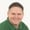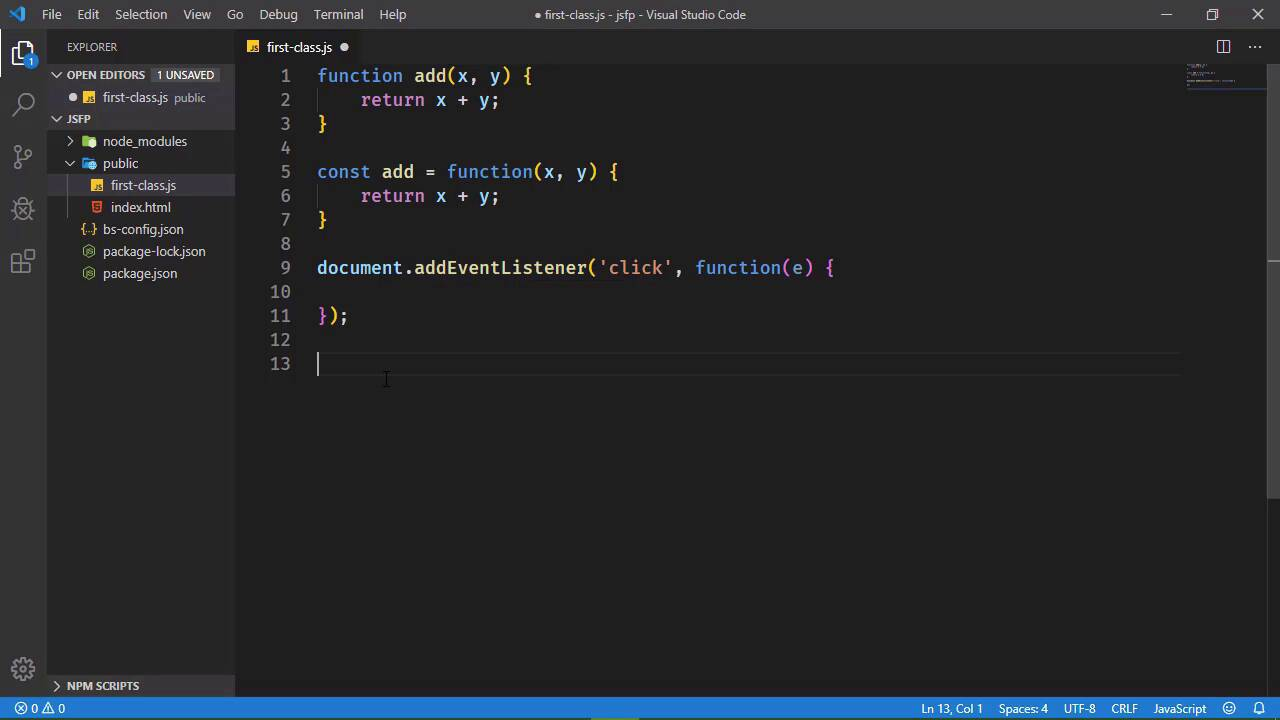FREELessons: 12Length: 1.1 hours• Overview
• Transcript

# 2.1 First-Class Functions

In JavaScript, functions are first-class objects. They are a type of data we can assign to variables, pass to other functions, and even return functions from within another function. It's a powerful feature and is central to functional programming.

## 4.Conclusion1 lesson, 00:48

### 2.1 First-Class Functions

Functions are very important to functional programming. I mean, that kinda goes without saying, doesn't it? But in JavaScript functions are what are called first class objects. And you might have heard that term but not really known what it means. Well, it's very important actually because you can't really have functional programming without functions being a central part of the language, which is kind of what first class means. I mean it doesn't exactly but let's do this. Let's create a new file called first-class.js and let's define a function. Let's just call it add, we will accept an x and y for parameters and then we will simply return x and y. Very simple function but by just defining this function, we have created a function object called add. So we have a type of data called function. Just like we have string or Boolean or number, we have a function. And since we have a data type called function, that means that we create these function objects and we can use them just like any other type of object in JavaScript. So that actually means that we can create a function and assign it to a variable. So you might see code that looks like this to where a function expression is being assigned to a variable. Now there is a slight difference between how the JavaScript engine actually interprets these two functions whenever it's loading it, but once it loads it, they are essentially identical. We end up with a function called add that accepts two values, and it performs a computation on those values. Now of course, we have two completely different function objections, this one that's defined with the declaration is completely different from this one that we defined with an expression. But that's just because we created two function objects that has nothing really to do with how they are created. So the idea that we can create a function and assign it to a variable, actually opens up a whole lot of doors that we normally wouldn't be able to do in some other languages. Like for example, we can pass a function as an argument to another function. Let's say that we wanted to set an event listener for the click event on the document object. Well, the first thing that we pass to add event listener is the type of event that we want to listen for. The second thing is the function. We are passing a function to another function and a functions were not first class objects in JavaScript. This probably wouldn't be possible. Well, it might be possible but not to the extent that it is. But if we can pass a function to another function that kind of also means that we can return a function from another function. For example, let's write a function called greet and there are really two parts to a greeting. There's the salutation, and then there's the name of the person that you're greeting. So the salutation could be Hello, hi, howdy or if you're from another part of the world, there's several different ways that you can say hello to somebody. So in this particular case, it would look very simple, in that we would have our salutation and then we would have the name after the salutation and that's all well and good. We've written functions like this all the time. But what if we wanted to write a more specialized function for greeting someone so that we would have like a function called howdy and then we would pass in the name Jim and so that would output howdy Jim or we would have hello and pass in Jim and that would be hello Jim. Well, we can write that in a couple of different ways but really the best way of doing that would be to write a function, that would be used for essentially building another function. So that our greet function would actually accept the salutation and then this function would return another function that accepted the name. And then of course, inside of this inner function, we would return the salutation and the name and I butchered that. There we go. So it required a little bit of extra code. But what this means is we could actually do something like this. We could create our howdy function by calling greet, and passing in howdy. And then all we would have to do is say howdy passing in Jim or Bob or whatever name that we wanted. And then if we wanted a function for using Hello, we would essentially do the same thing so that we would pass in Hello for the salutation and then we could use Hello for the same purpose. So what we end up with is actually a good way of reusing code. If we were going to write this using object oriented principles, we would have a greeter class that would have a property for the salutation and then it would have a method to actually perform the greeting. So the first thing that I want you to do, is to start thinking of functions in terms of being an object. Yes, it is something that we can execute, but more importantly, it's something that we can store within a variable. We can pass it to other functions. We can even return it from other functions. Because all of the ideas and concepts that go into functional programming, all revolve around that.

Back to the top